﻿ 充液系统中液体晃动的全局分岔和混沌

# 充液系统中液体晃动的全局分岔和混沌Global Bifurcation and Chaos of Liquid Sloshing in Liquid-Filled System

Abstract: The nonlinear governing equations of the liquid sloshing modals in cylindrical storage tank are established. Through integration, the analytical expressions of the homoclinic orbits of this kind of system are got. Using the Melnikov method, the global dynamical behaviors are analyzed. The threshold of the chaotic motion is calculated, which shows that there exists global bifurcation and chaos when the system is subjected to greater disturbance. Meanwhile, numerical simulations are also given, which confirm the analytical results.

1. 引言

2. 模型的建立

$\frac{\partial \Phi }{\partial t}+\frac{1}{2}{\left(\nabla \Phi -\stackrel{˙}{U}-\Omega ×r\right)}^{2}-\frac{1}{2}{\left(\Omega ×r\right)}^{2}+\Pi \mp \frac{2\sigma \stackrel{¯}{H}}{\rho }=0$ (1)

${\stackrel{¨}{\eta }}_{m}+2{\varsigma }_{m}{\stackrel{˙}{\eta }}_{m}+{\upsilon }_{m}^{2}{\eta }_{m}+\underset{i=1}{\overset{N}{\sum }}\underset{j=1}{\overset{N}{\sum }}{\sigma }_{ijm}{\eta }_{i}{\eta }_{j}+\underset{i=1}{\overset{N}{\sum }}\underset{j=1}{\overset{N}{\sum }}\underset{k=1}{\overset{N}{\sum }}{\sigma }_{ijkm}{\eta }_{i}{\eta }_{j}{\eta }_{k}+{\stackrel{˜}{\alpha }}_{m}\stackrel{¨}{X}+{\stackrel{˜}{e}}_{m}\stackrel{¨}{Y}=0,\text{\hspace{0.17em}}$ $m=1,2,3,\cdots$ (2)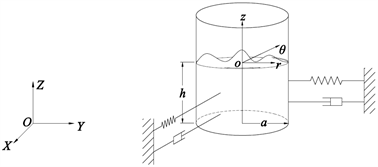Figure 1. System model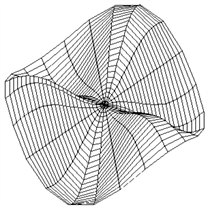Figure 2. Modal of sloshing liquid

${\stackrel{¨}{\eta }}_{1}+{\upsilon }_{1}^{2}{\eta }_{1}={K}_{31}{\stackrel{˙}{\eta }}_{1}+{K}_{32}{\eta }_{1}^{2}+{K}_{33}{\eta }_{1}^{3}+{K}_{34}\mathrm{cos}\left({\Omega }_{1}t\right)$ (3)

3. 全局分岔

$\stackrel{¨}{f}+{\lambda }_{1}f+{\lambda }_{2}{f}^{2}+{\lambda }_{3}{f}^{3}=\epsilon \left(g\mathrm{cos}\omega t-\delta \stackrel{˙}{f}\right)$ (4)

$\left\{\begin{array}{c}y=\stackrel{˙}{f}\\ \stackrel{˙}{y}=-{\lambda }_{1}f-{\lambda }_{2}{f}^{2}-{\lambda }_{3}{f}^{3}\end{array}$ (5)

$H=\frac{1}{2}{\stackrel{˙}{f}}^{2}+\frac{1}{2}{\lambda }_{1}{f}^{2}+\frac{1}{3}{\lambda }_{2}{f}^{3}+\frac{1}{4}{\lambda }_{3}{f}^{4}$ (6)

${\stackrel{˙}{f}}^{*}=±\sqrt{-{\lambda }_{1}{f}^{*2}-\frac{2}{3}{\lambda }_{2}{f}^{*3}-\frac{1}{2}{\lambda }_{3}{f}^{*4}}$ (7)

$-\frac{1}{\sqrt{-{\lambda }_{1}}}\mathrm{ln}|\frac{\sqrt{-{\lambda }_{1}-\frac{2}{3}{\lambda }_{2}{f}^{*}-\frac{1}{2}{\lambda }_{3}{f}^{*2}}+\sqrt{-{\lambda }_{1}}}{{f}^{*}}-\frac{{\lambda }_{2}}{3\sqrt{-{\lambda }_{1}}}|={C}_{1}±t$ (8)

$|\frac{\sqrt{-{\lambda }_{1}-\frac{2}{3}{\lambda }_{2}{f}^{*}-\frac{1}{2}{\lambda }_{3}{f}^{*2}}+\sqrt{-{\lambda }_{1}}}{{f}^{*}}-\frac{{\lambda }_{2}}{3\sqrt{-{\lambda }_{1}}}|={C}_{2}{\text{e}}^{±\sqrt{-{\lambda }_{1}t}}$ (9)

$H<0$ 时，得绕O、A两点的两族闭轨道，代表在稳定平衡位置附近的振动，当H增大时，振动的周期增大，当 $H>0$ 时，代表另一类型的周期性振动。

$M\left(\tau \right)={\int }_{-\infty }^{+\infty }{\stackrel{˙}{f}}^{*}\varphi \left[{f}^{*},{\stackrel{˙}{f}}^{*},f\omega \left(t+\tau \right)\right]\text{d}t$ (10)

$M\left(\tau \right)=-\delta {\int }_{-\infty }^{+\infty }{\stackrel{˙}{f}}^{*2}\text{d}t+g{\int }_{-\infty }^{+\infty }{\stackrel{˙}{f}}^{*}\mathrm{cos}\omega \left(t+\tau \right)\text{d}t$ (11)

${\int }_{-\infty }^{+\infty }{\stackrel{˙}{f}}^{*2}\text{d}t=\frac{4}{3{\lambda }_{3}}\left[{\left(-{\lambda }_{1}\right)}^{\frac{3}{2}}+\frac{{\lambda }_{2}^{2}\sqrt{-{\lambda }_{1}}}{3{\lambda }_{3}}+{\lambda }_{2}\frac{2{\lambda }_{2}^{2}-9{\lambda }_{1}{\lambda }_{3}}{9{\lambda }_{3}\sqrt{2{\lambda }_{3}}}\mathrm{arcsin}\frac{\sqrt{2}{\lambda }_{2}}{\sqrt{2{\lambda }_{2}^{2}-9{\lambda }_{1}{\lambda }_{3}}}\right]$ (12)

$\begin{array}{c}{\int }_{-\infty }^{+\infty }{\stackrel{˙}{f}}^{*}\mathrm{cos}\omega \left(t+\tau \right)\text{d}t={\int }_{-\infty }^{+\infty }\mathrm{cos}\omega \left(t+\tau \right)\text{d}{f}^{*}\\ =2{C}_{2}\sqrt{{\lambda }_{1}}\omega {\int }_{-\infty }^{+\infty }\frac{{\text{e}}^{\sqrt{{\lambda }_{1}}t}\mathrm{sin}\omega \left(t+\tau \right)}{{\left({C}_{2}{\text{e}}^{\sqrt{{\lambda }_{1}}t}+\frac{{\lambda }_{2}}{3\sqrt{{\lambda }_{1}}}\right)}^{2}+\frac{1}{2}{\lambda }_{3}}\text{d}t\end{array}$ (13)

${\int }_{-\infty }^{+\infty }\frac{{\text{e}}^{\sqrt{{\lambda }_{1}}t}\mathrm{sin}\omega \left(t+\tau \right)}{{\left({C}_{2}{\text{e}}^{\sqrt{{\lambda }_{1}}t}+\frac{{\lambda }_{2}}{3\sqrt{{\lambda }_{1}}}\right)}^{2}+\frac{1}{2}{\lambda }_{3}}\text{d}t=2\text{π}\cdot \frac{\mathrm{sin}\omega \left(\xi +\tau \right)\left(ch\omega {\psi }_{2}-ch\omega {\psi }_{1}\right)}{{C}_{2}\sqrt{2{\lambda }_{1}{\lambda }_{3}}\left(1-ch\frac{2\text{π}\omega }{\sqrt{{\lambda }_{1}}}\right)}$ (14)

$\left\{\begin{array}{l}\xi =\frac{1}{\sqrt{{\lambda }_{1}}}\mathrm{ln}\left[\frac{1}{3{C}_{2}}\sqrt{\frac{2{\lambda }_{2}^{2}+9{\lambda }_{1}{\lambda }_{3}}{2{\lambda }_{1}}}\right]\\ {\psi }_{1}=\frac{1}{\sqrt{{\lambda }_{1}}}\left[\text{π}+\text{arctg}\left(\frac{3}{{\lambda }_{2}}\sqrt{\frac{{\lambda }_{1}{\lambda }_{3}}{2}}\right)\right]\\ {\psi }_{2}=\frac{1}{\sqrt{{\lambda }_{1}}}\left[\text{π}-\text{arctg}\left(\frac{3}{{\lambda }_{2}}\sqrt{\frac{{\lambda }_{1}{\lambda }_{3}}{2}}\right)\right]\end{array}$ (15)

$\begin{array}{l}M\left(\tau \right)=-\frac{4\delta }{3{\lambda }_{3}}\left[{\lambda }_{1}^{\frac{3}{2}}+\frac{{\lambda }_{2}^{2}\sqrt{{\lambda }_{1}}}{3{\lambda }_{3}}+{\lambda }_{2}\cdot \frac{2{\lambda }_{2}^{2}+9{\lambda }_{1}{\lambda }_{3}}{9{\lambda }_{3}\sqrt{2{\lambda }_{3}}}\mathrm{arcsin}\frac{\sqrt{2}{\lambda }_{2}}{\sqrt{2{\lambda }_{2}^{2}+9{\lambda }_{1}{\lambda }_{3}}}\right]\\ \text{ }\text{ }\text{ }\text{\hspace{0.17em}}+\frac{4\text{π}g\omega \mathrm{sin}\omega \left(\xi +\tau \right)\left(ch\omega {\psi }_{1}-ch\omega {\psi }_{2}\right)}{\sqrt{2{\lambda }_{3}}\left(ch\frac{2\text{π}\omega }{\sqrt{{\lambda }_{1}}}-1\right)}\end{array}$ (16)

Melnikov函数如果有限，则稳定流形和不稳定流形相交，Poincare映射有马蹄，从而存在着奇怪的不变集合，对我们研究的耗散系统，就有可能出现混沌，由 $M\left(\tau \right)=0$ 得到

$\mathrm{sin}\omega \left(\xi +\tau \right)=\frac{\delta \left(ch\frac{2\text{π}\omega }{\sqrt{{\lambda }_{1}}}-1\right)}{6\sqrt{2}g\omega \sqrt{{\lambda }_{3}}\left(ch\omega {\psi }_{1}-ch\omega {\psi }_{2}\right)}\left[{\lambda }_{1}^{\frac{3}{2}}+\frac{{\lambda }_{2}^{2}\sqrt{{\lambda }_{1}}}{3{\lambda }_{3}}+{\lambda }_{2}\cdot \frac{2{\lambda }_{2}^{2}+9{\lambda }_{1}{\lambda }_{3}}{9{\lambda }_{3}\sqrt{2{\lambda }_{3}}}\mathrm{arcsin}\frac{\sqrt{2}{\lambda }_{2}}{\sqrt{2{\lambda }_{2}^{2}+9{\lambda }_{1}{\lambda }_{3}}}\right]$ (17)

$\frac{g}{\delta }\ge \frac{\left(ch\frac{2\text{π}\omega }{\sqrt{{\lambda }_{1}}}-1\right)}{6\sqrt{2}\omega \sqrt{{\lambda }_{3}}\left(ch\omega {\psi }_{1}-ch\omega {\psi }_{2}\right)}\left[{\lambda }_{1}^{\frac{3}{2}}+\frac{{\lambda }_{2}^{2}\sqrt{{\lambda }_{1}}}{3{\lambda }_{3}}+{\lambda }_{2}\cdot \frac{2{\lambda }_{2}^{2}+9{\lambda }_{1}{\lambda }_{3}}{9{\lambda }_{3}\sqrt{2{\lambda }_{3}}}\mathrm{arcsin}\frac{\sqrt{2}{\lambda }_{2}}{\sqrt{2{\lambda }_{2}^{2}+9{\lambda }_{1}{\lambda }_{3}}}\right]=R\left(\omega \right)$ (18)

4. 算例

5. 结论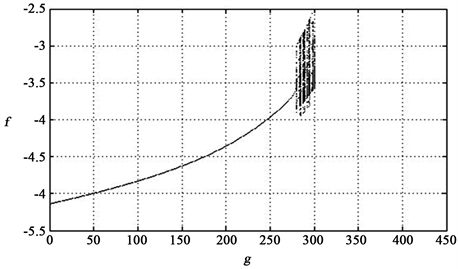Figure 3. Bifurcation diagram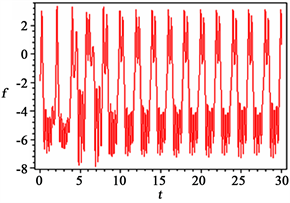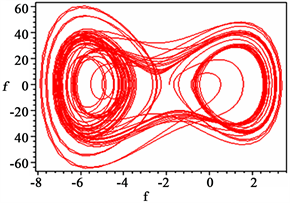(a) (b)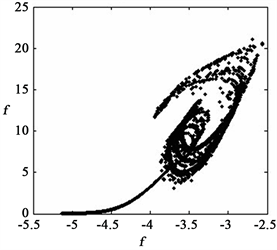(c)

Figure 4. Curves of the responses at g = 280 (a) Time history; (b) Phase plane; (c) Poincare mapping

 Abramson, H.N. (2003) Dynamics of Contained Liquids: A Personal Odyssey. Applied Mechanics Review, 56, 1-7.
https://doi.org/10.1115/1.1511517

 Ibrahim, R.A. and Pilipchuk, V.N. (2001) Recent Advances in Liquid Sloshing Dynamics. Applied Mechanics Review, 54, 133-199.
https://doi.org/10.1115/1.3097293

 Pan, X. and Jin, W. (2005) Effect of Axial Vibration on Free Surface Flows in Cylindrical Liquid. Chinese Physics B, 14, 422-426.
https://doi.org/10.1088/1009-1963/14/2/035

 王照林, 刘延柱. 充液系统动力学[M]. 北京: 科学出版社, 2002: 83-98.

 Zhou, L., Chen, Y. and Chen, F. (2009) Stability and Chaos of a Damped Satellite Partially Filled with Liquid. Acta Astronautica, 65, 1628-1638.
https://doi.org/10.1016/j.actaastro.2009.04.009

 Askari, E. and Daneshmand, F. (2009) Coupled Vibration of a Partially Fluid-Filled Cylindrical Container with a Cylindrical Internal Body. Journal of Fluids and Structures, 25, 389-405.
https://doi.org/10.1016/j.jfluidstructs.2008.07.003

 Liu, Y., Zhu, J. and Luo, X. (2009) Bifurcation and Stabil-ity of an Improved Time-Delayed Fluid Flow Model in Internet Congestion Control. Chinese Physics B, 18, 3772-3776.
https://doi.org/10.1088/1674-1056/18/9/026

 尹立中, 马兴瑞, 王本利, 邹经湘. 圆柱贮箱类液固耦合系统转动响应分析[J]. 振动工程学报, 2001, 14(1): 68-71.

 马兴瑞, 王本利, 苟兴宇. 航天器动力学－若干问题进展及应用[M]. 北京: 科学出版社, 2001: 329-335.

 Shi, P., Han, D. and Liu, B. (2010) Chaos and Chaotic Con-trol in a Relative Rotation Nonlinear Dynamical System under Parametric Excitation. Chinese Physics B, 19, Article ID: 090306.

 Lv, L., Li, G., Guo, L., Meng, L., Zou, J. and Yang, M. (2010) Generalized Chaos Synchronization of a Weighted Complex Network with Different Nodes. Chinese Physics B, 19, Article ID: 080507.

 Kovaleva, A. (2010) The Melnikov Criterion of Instability for Random Rocking Dynamics of a Rigid Block with an Attached Secondary Structure. Nonlinear Analysis: Real World Applications, 11, 472-479.
https://doi.org/10.1016/j.nonrwa.2008.12.001

 Rasoul, A. and Hamid, R.Z.Z. (2010) Bifurcations of Limit Cy-cles for a Quintic Hamiltonian System with a Double Cuspidal Loop. Computers & Mathematics with Applications, 59, 1409-1418.
https://doi.org/10.1016/j.camwa.2009.12.024

 Zhou, L., Chen, Y. and Chen, F. (2010) Global Bifurcation Analysis and Chaos of an Arch Structure with Parametricand Forced Excitation. Mechanics Research Communications, 37, 67-71.
https://doi.org/10.1016/j.mechrescom.2009.08.007

 Zhang, W., Zhang, J.H., Yao, M.H. and Yao, Z.G. (2010) Multi-Pulse Chaotic Dynamics of Non-Autonomous Nonlinear System for a Laminated Composite Piezoelectric Rectan-gular Plate. Acta Mechanica, 211, 23-47.
https://doi.org/10.1007/s00707-009-0210-3

 Zhong, S. and Chen, Y. (2011) Bifurcation of Elastic Tank-Liquid Coupled Sloshing System. Applied Mathematics and Mechanics, 32, 1169-1176.
https://doi.org/10.1007/s10483-011-1490-x

 Chen, Y., Audrew, Y. and Leung, T. (1998) Bifurcation and Chaos in Engineering. Springe-Vereag, London, 66-76.
https://doi.org/10.1007/978-1-4471-1575-5

Top